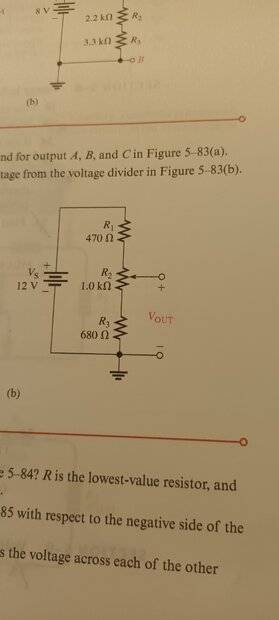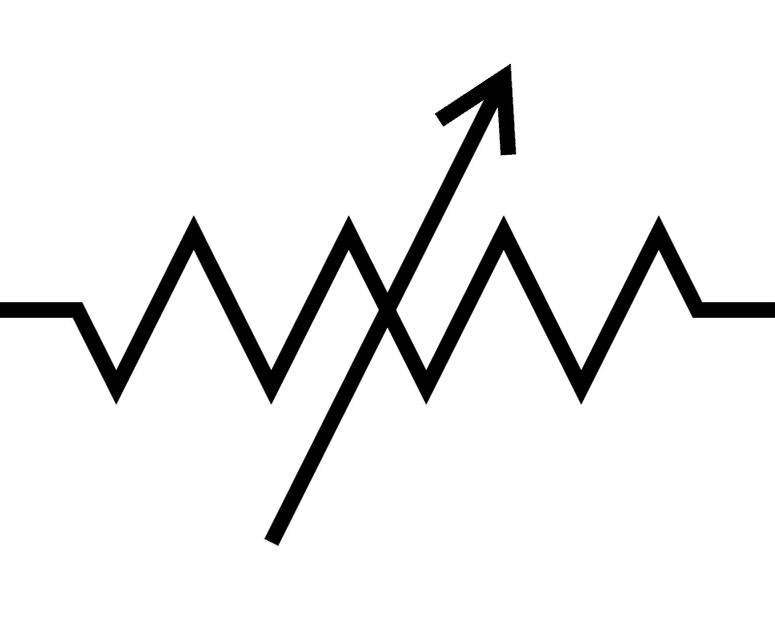# Voltage Variation w/ Variable Resistor: Correct?

• Lay1

#### Lay1

Homework Statement
Determine the minimum and maximum voltage from the voltage divider in the figure.
Relevant Equations
V=IR
V(drop)=Rx/Rt * VsIn this figure, I suppose the maximum voltage is when R2=1kohm and the minimum voltage is when R2=0kohm, which means R2 is a variable resistor. Is the way I think is correct or not? Please give me suggestions. Thank you.

No. The value of R2 doesn't change. What changes is the portion of the voltage drop that is above or below the tap point where Vout measured. Imagine that R2 is a bar of resistive material, and you are changing the location of your voltmeter + probe along that bar. One extreme has the probe at the R3 end, the other is at the R2 end, but the resistors and currents don't change.

•TSny
The variable resistor you described would be drawn like this in a schematic. It only has two connections, and it's value does change from 0 to the indicated value.No. The value of R2 doesn't change. What changes is the portion of the voltage drop that is above or below the tap point where Vout measured. Imagine that R2 is a bar of resistive material, and you are changing the location of your voltmeter + probe along that bar. One extreme has the probe at the R3 end, the other is at the R2 end, but the resistors and currents don't change.
So, what you mean is that the voltmeter measures with R2 for maximum voltage drop and for minimum, without R2, but for R3. In all cases, R2 is not considered as a variable resistor. Thank you for your precious reply.

•DaveE
The variable resistor you described would be drawn like this in a schematic. It only has two connections, and it's value does change from 0 to the indicated value.
View attachment 324752
Yes, thank you for your information.